Скачать презентацию Lattice-based Fault Attacks on DSA Another Possible

768c75226a206a57dde70979b0301285.ppt

• Количество слайдов: 15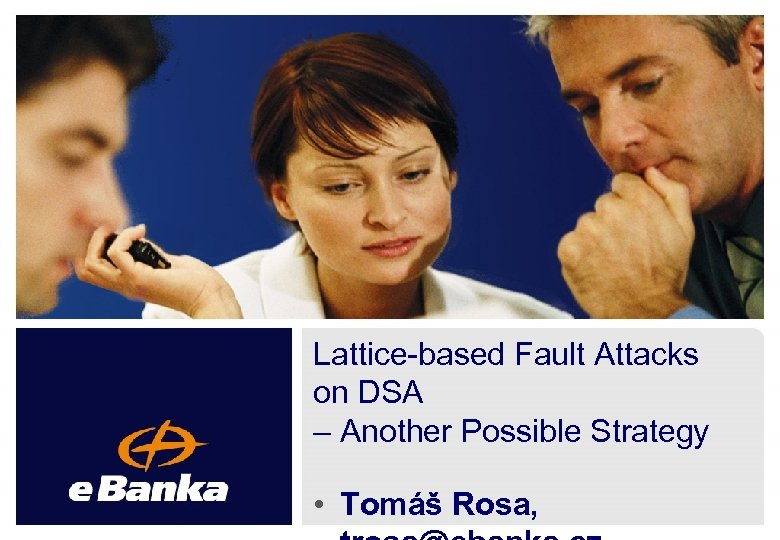Lattice-based Fault Attacks on DSA – Another Possible Strategy • Tomáš Rosa,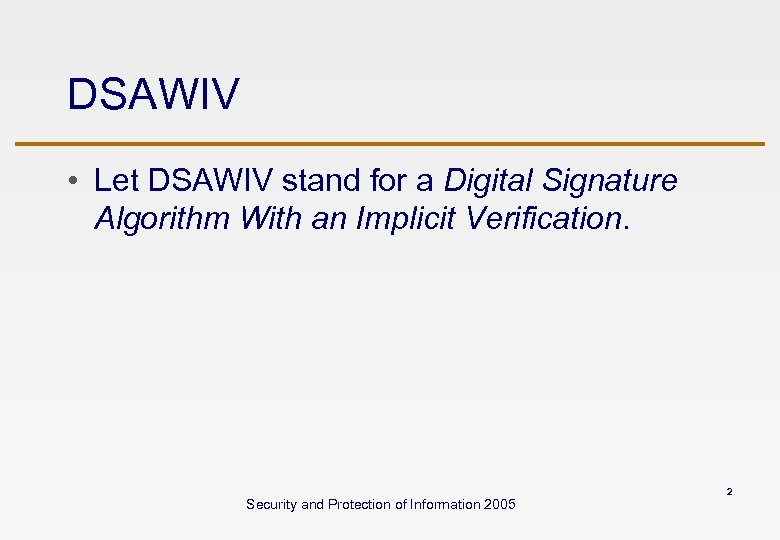DSAWIV • Let DSAWIV stand for a Digital Signature Algorithm With an Implicit Verification. Security and Protection of Information 2005 2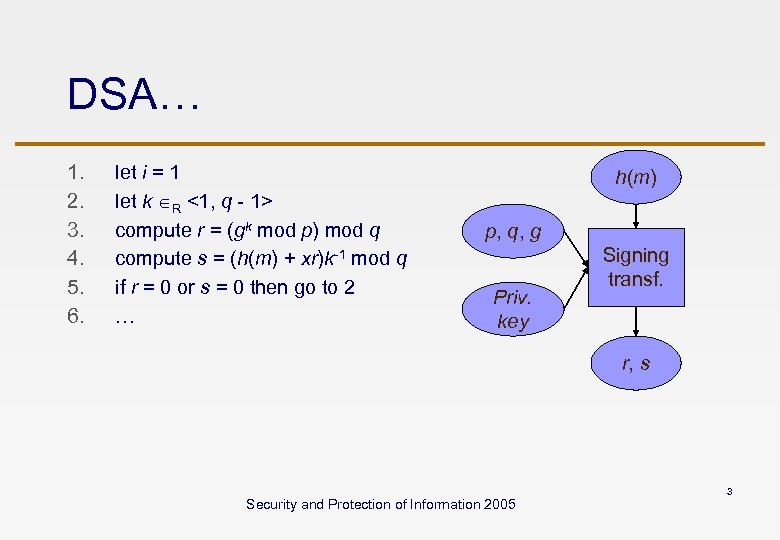DSA… 1. 2. 3. 4. 5. 6. let i = 1 let k R <1, q - 1> compute r = (gk mod p) mod q compute s = (h(m) + xr)k-1 mod q if r = 0 or s = 0 then go to 2 … h(m) p, q, g Priv. key Signing transf. r, s Security and Protection of Information 2005 3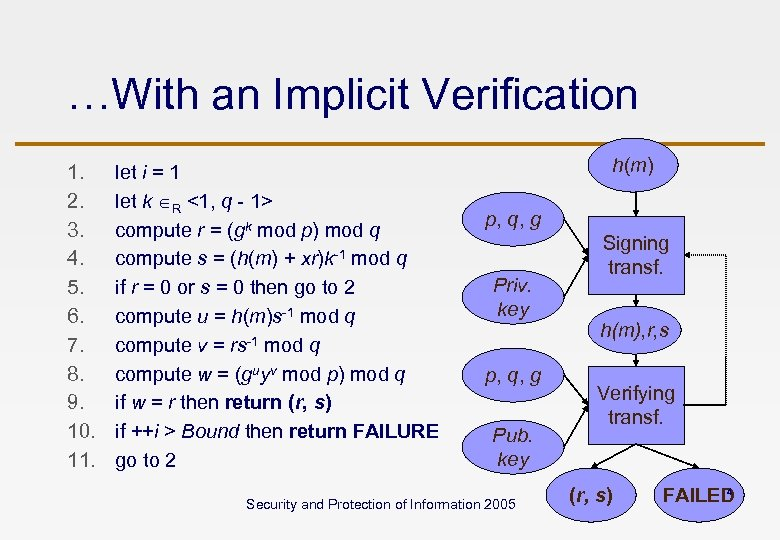…With an Implicit Verification 1. 2. 3. 4. 5. 6. 7. 8. 9. 10. 11. let i = 1 let k R <1, q - 1> compute r = (gk mod p) mod q compute s = (h(m) + xr)k-1 mod q if r = 0 or s = 0 then go to 2 compute u = h(m)s-1 mod q compute v = rs-1 mod q compute w = (guyv mod p) mod q if w = r then return (r, s) if ++i > Bound then return FAILURE go to 2 h(m) p, q, g Priv. key p, q, g Pub. key Security and Protection of Information 2005 Signing transf. h(m), r, s Verifying transf. (r, s) 4 FAILED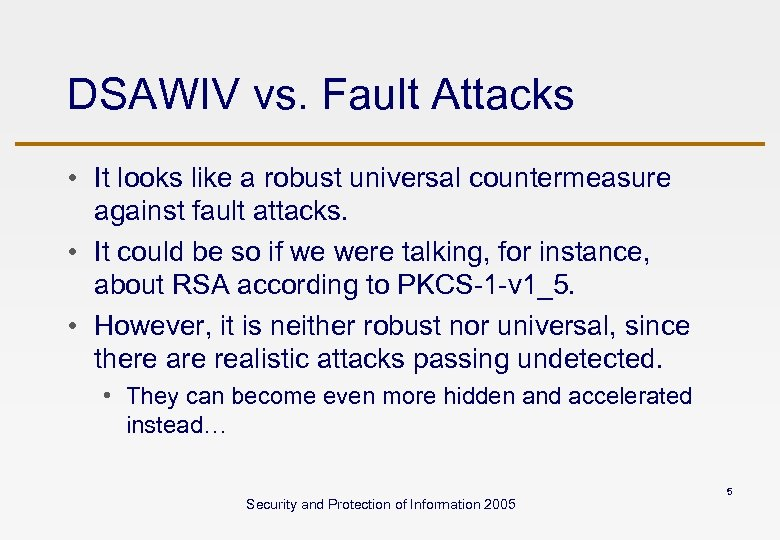DSAWIV vs. Fault Attacks • It looks like a robust universal countermeasure against fault attacks. • It could be so if we were talking, for instance, about RSA according to PKCS-1 -v 1_5. • However, it is neither robust nor universal, since there are realistic attacks passing undetected. • They can become even more hidden and accelerated instead… Security and Protection of Information 2005 5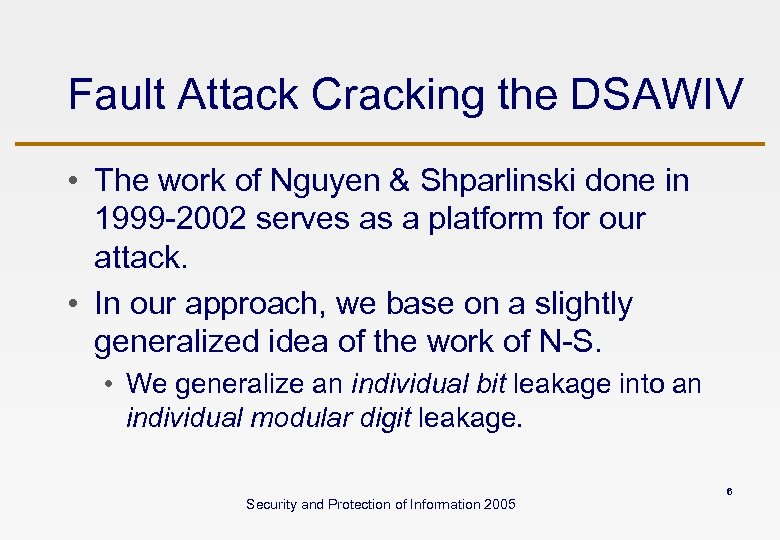Fault Attack Cracking the DSAWIV • The work of Nguyen & Shparlinski done in 1999 -2002 serves as a platform for our attack. • In our approach, we base on a slightly generalized idea of the work of N-S. • We generalize an individual bit leakage into an individual modular digit leakage. Security and Protection of Information 2005 6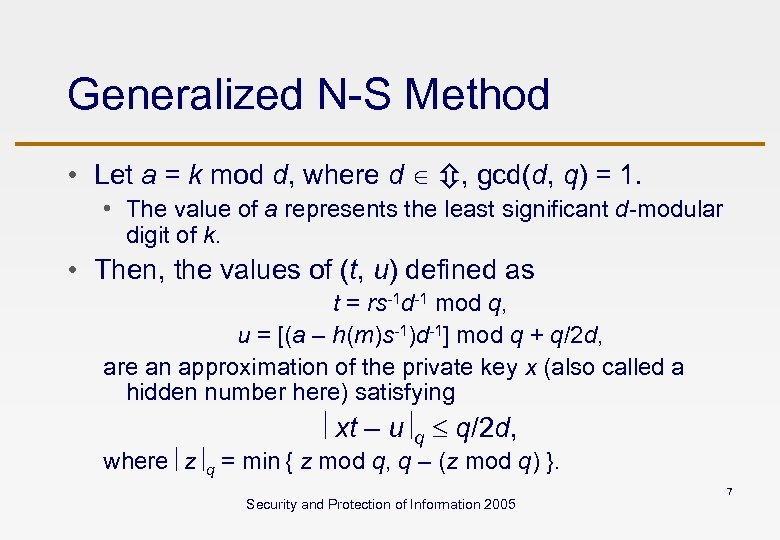Generalized N-S Method • Let a = k mod d, where d , gcd(d, q) = 1. • The value of a represents the least significant d-modular digit of k. • Then, the values of (t, u) defined as t = rs-1 d-1 mod q, u = [(a – h(m)s-1)d-1] mod q + q/2 d, are an approximation of the private key x (also called a hidden number here) satisfying xt – u q q/2 d, where z q = min { z mod q, q – (z mod q) }. Security and Protection of Information 2005 7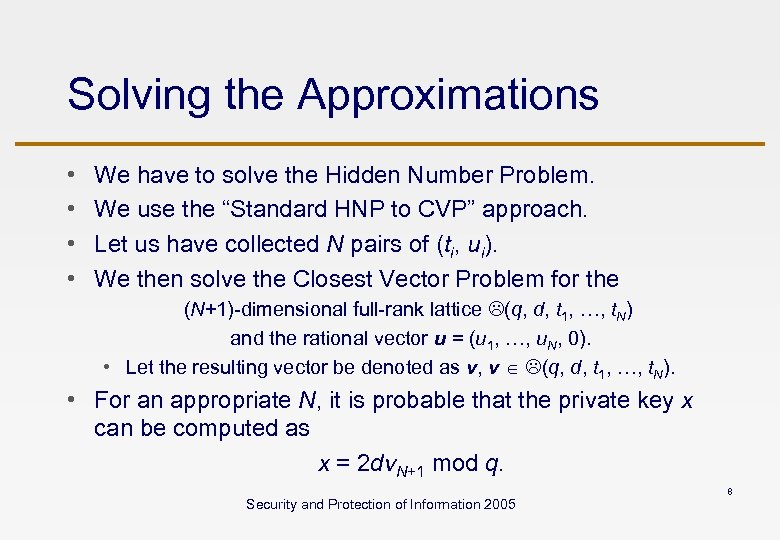Solving the Approximations • • We have to solve the Hidden Number Problem. We use the “Standard HNP to CVP” approach. Let us have collected N pairs of (ti, ui). We then solve the Closest Vector Problem for the (N+1)-dimensional full-rank lattice (q, d, t 1, …, t. N) and the rational vector u = (u 1, …, u. N, 0). • Let the resulting vector be denoted as v, v (q, d, t 1, …, t. N). • For an appropriate N, it is probable that the private key x can be computed as x = 2 dv. N+1 mod q. Security and Protection of Information 2005 8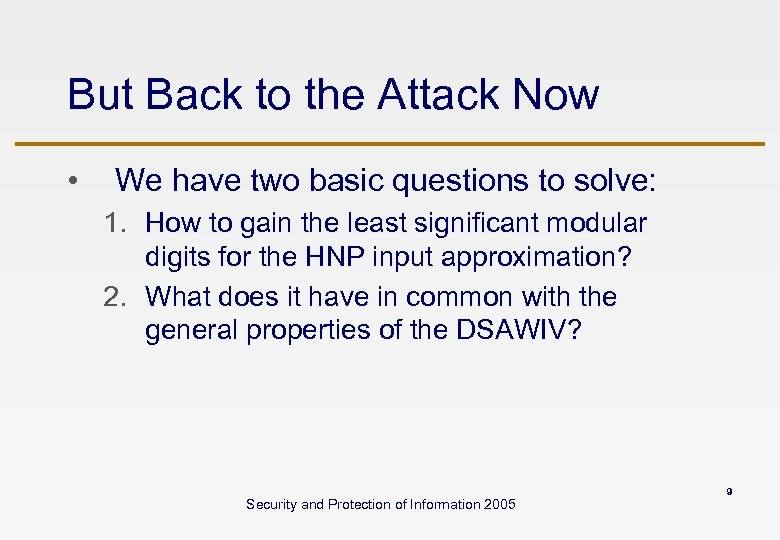But Back to the Attack Now • We have two basic questions to solve: 1. How to gain the least significant modular digits for the HNP input approximation? 2. What does it have in common with the general properties of the DSAWIV? Security and Protection of Information 2005 9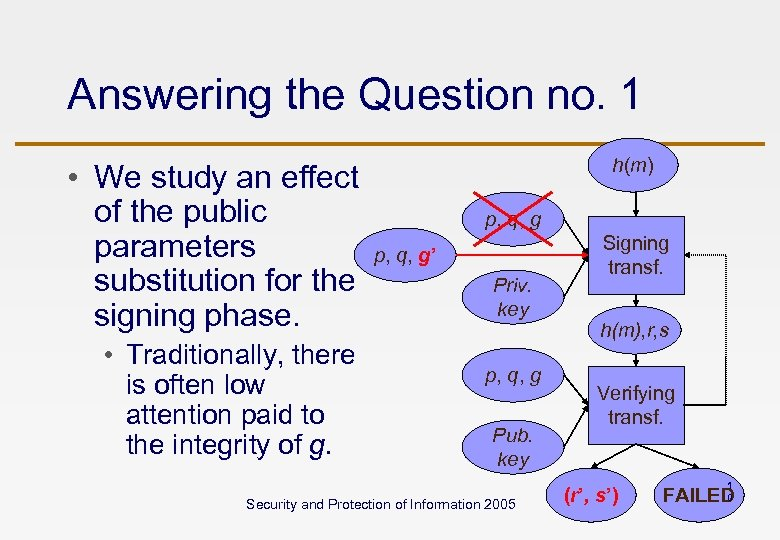Answering the Question no. 1 • We study an effect of the public parameters substitution for the signing phase. • Traditionally, there is often low attention paid to the integrity of g. h(m) p, q, g’ Priv. key p, q, g Pub. key Security and Protection of Information 2005 Signing transf. h(m), r, s Verifying transf. (r’, s’) 1 0 FAILED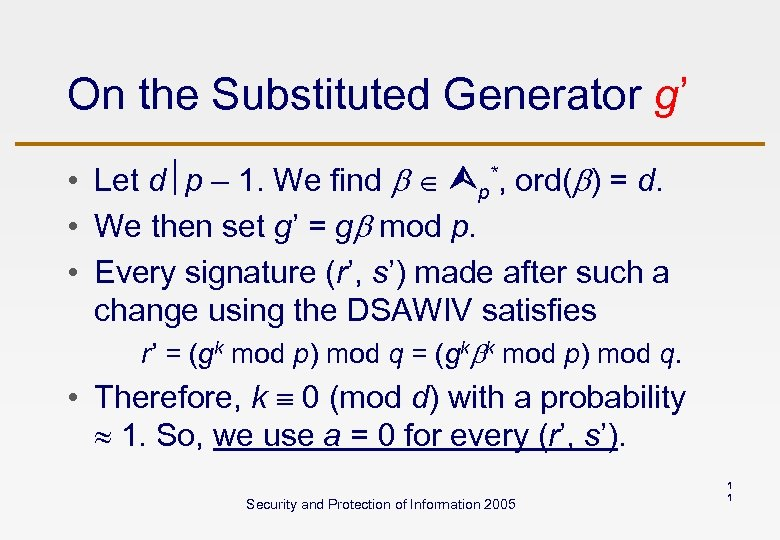On the Substituted Generator g’ • Let d p – 1. We find p*, ord( ) = d. • We then set g’ = g mod p. • Every signature (r’, s’) made after such a change using the DSAWIV satisfies r’ = (gk mod p) mod q = (gk k mod p) mod q. • Therefore, k 0 (mod d) with a probability 1. So, we use a = 0 for every (r’, s’). Security and Protection of Information 2005 1 1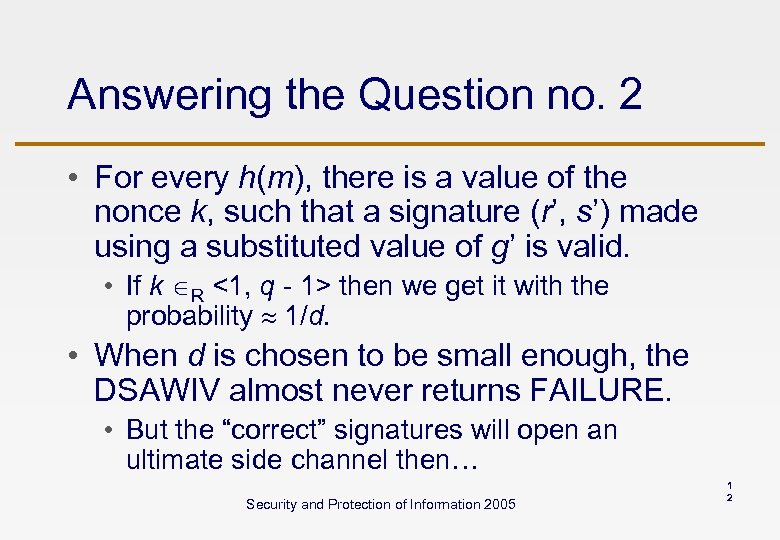Answering the Question no. 2 • For every h(m), there is a value of the nonce k, such that a signature (r’, s’) made using a substituted value of g’ is valid. • If k R <1, q - 1> then we get it with the probability 1/d. • When d is chosen to be small enough, the DSAWIV almost never returns FAILURE. • But the “correct” signatures will open an ultimate side channel then… Security and Protection of Information 2005 1 2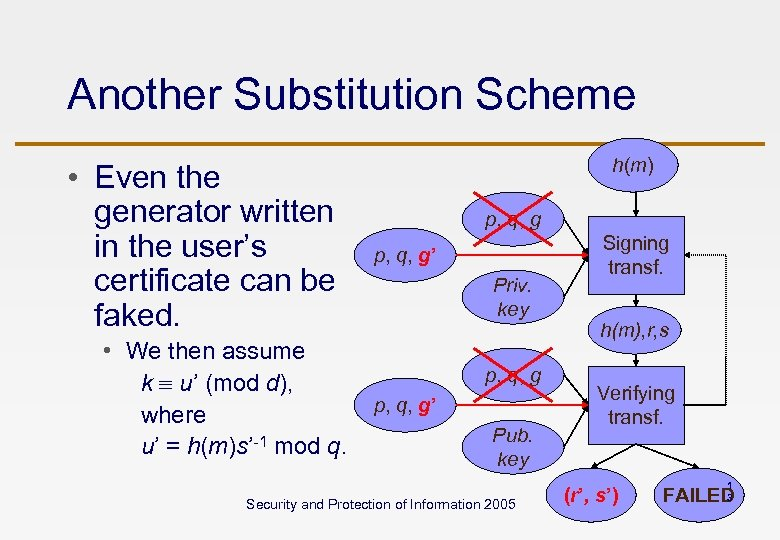Another Substitution Scheme • Even the generator written in the user’s certificate can be faked. • We then assume k u’ (mod d), where u’ = h(m)s’-1 mod q. h(m) p, q, g’ Priv. key p, q, g’ Pub. key Security and Protection of Information 2005 Signing transf. h(m), r, s Verifying transf. (r’, s’) 1 3 FAILED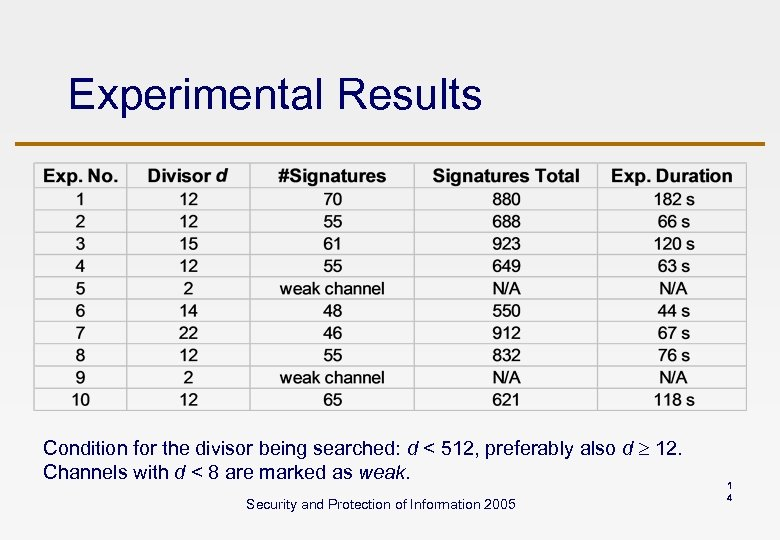Experimental Results Condition for the divisor being searched: d < 512, preferably also d 12. Channels with d < 8 are marked as weak. Security and Protection of Information 2005 1 4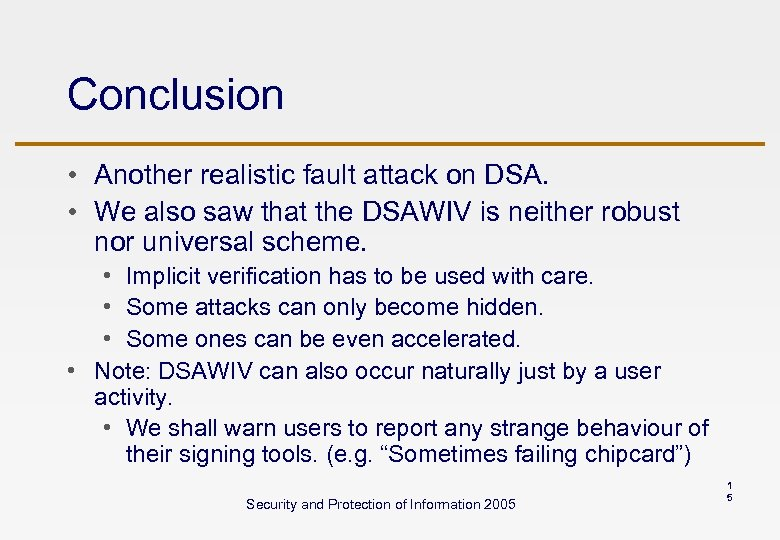Conclusion • Another realistic fault attack on DSA. • We also saw that the DSAWIV is neither robust nor universal scheme. • Implicit verification has to be used with care. • Some attacks can only become hidden. • Some ones can be even accelerated. • Note: DSAWIV can also occur naturally just by a user activity. • We shall warn users to report any strange behaviour of their signing tools. (e. g. “Sometimes failing chipcard”) Security and Protection of Information 2005 1 5